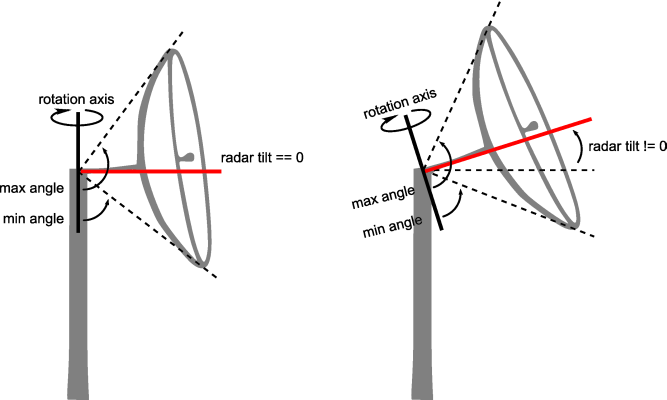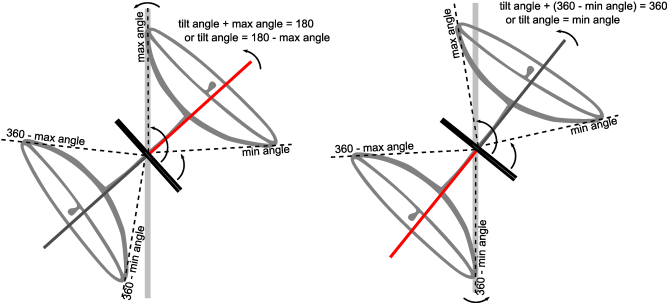2023.0.07

• All Superinterfaces:
ILcdLOSPropagationFunction
All Known Implementing Classes:

```public interface ILcdLOSRadarPropagationFunction
extends ILcdLOSPropagationFunction```
Extension of the interface `ILcdLOSPropagationFunction` representing a radar line-of-sight propagation function. It adds some property methods that can be used by the propagation functions which are based on a radar line-of-sight computation.

The matrix resulting from this propagation function can contain the following special values:

The following image gives a visual explanation of the parameters of a radar propagation function. The thin black arrows denote the minimum and maximum vertical angles. The red line corresponds to the radar tilt angle. The whole radar is rotated around the rotation axis.Keep in mind that, if the radar tilt angle is not zero, the minimum and maximum viewing angles should be recomputed in the method `ILcdLOSPropagationFunction.applyForAzimuthSFCT(double, ILcdLOSCoverageMatrix)`. In some cases, the computed viewing angle might fall outside the viewing interval [0, 180]. The implementation of this interface should handle these special cases correctly. The viewing angle is usually clipped to `0` or `180`.

There are two cases when a viewing angle can fall outside the viewing interval. They are both explained using the image below. Both drawings show on the top-right part the viewing angles as dotted lines for the azimuth `aMaxRadarTiltAzimuth` and on the bottom-left part for the azimuth `aMaxRadarTiltAzimuth + 180`. The red lines correspond to the radar tilt angle for the azimuth.

• case 1: when the maximum viewing angle becomes larger than `180` degrees. In the left drawing, the radar tilt angle is chosen to make the maximum viewing angle fall on the `180` degree line. A larger radar tilt angle makes the maximum viewing angle fall outside the viewing interval [0, 180].
• case 2: when the minimum viewing angle becomes smaller than `0` degrees. In the right drawing, the radar tilt angle is chosen to make the minimum viewing angle fall on the `0` degree line. Larger radar tilt angles make the minimum viewing angle fall outside the viewing interval [0, 180].Note that these cases are only an issue when the corresponding azimuths lies inside the area on which this radar propagation function will be applied. This area is defined by the line-of-sight coverage (`TLcdLOSCoverage`).

`TLcdLOSRadarPropagationFunction`, `TLcdLOSRadarPropagationFunctionSkyBackground`
• ### Field Summary

Fields
Modifier and Type Field and Description
`static int` `INVISIBLE`
The resulting value for points where the object is invisible.
`static int` `UNKNOWN`
The resulting value for points where the visibility is unknown.
• ### Method Summary

All Methods
Modifier and Type Method and Description
`TLcdCoverageAltitudeMode` `getAltitudeMode()`
Returns the altitude mode indicating the definition of the resulting line-of-sight values.
`TLcdEarthRepresentationMode` `getEarthRepresentationMode()`
Returns the earth representation mode used by this instance.
`double` `getKFactor()`
Returns the K-factor indicating the reflection of radar waves on the atmosphere.
`double` `getMaxRadarTiltAngle()`
Returns the maximum radar tilt angle in degrees, starting from 0 at the horizontal plane to 90 at zenith.
`double` `getMaxRadarTiltAzimuth()`
Returns the azimuth in degrees where the maximum radar tilt angle occurs.
`double` `getMaxVerticalAngle()`
Returns the maximum angle of the vertical extent in degrees, starting from 0 at the ground to 180 at zenith.
`double` `getMinVerticalAngle()`
Returns the minimum angle of the vertical extent in degrees, starting from 0 at the ground to 180 at zenith.
`ALcdTerrainElevationProvider` `getTerrainElevationProvider()`
Returns the elevation provider used to retrieve the terrain elevation data.
• ### Methods inherited from interface com.luciad.tea.ILcdLOSPropagationFunction

`applyForAzimuthSFCT, createLOSCoverageMatrix, initialize`
• ### Field Detail

• #### INVISIBLE

`static final int INVISIBLE`
The resulting value for points where the object is invisible.
Constant Field Values
• #### UNKNOWN

`static final int UNKNOWN`
The resulting value for points where the visibility is unknown. For example when no elevation data or invalid data was found at that point.
Constant Field Values
• ### Method Detail

• #### getEarthRepresentationMode

`TLcdEarthRepresentationMode getEarthRepresentationMode()`
Returns the earth representation mode used by this instance.
Returns:
the earth representation mode used by this instance.
• #### getAltitudeMode

`TLcdCoverageAltitudeMode getAltitudeMode()`
Returns the altitude mode indicating the definition of the resulting line-of-sight values.
Returns:
the altitude mode indicating the definition of the resulting line-of-sight values.
• #### getKFactor

`double getKFactor()`
Returns the K-factor indicating the reflection of radar waves on the atmosphere. For radar computations, values should be greater than 1, usually 4/3. Setting the value equal to 1 will yield the same results as visual line-of-sight computations.
Returns:
the K-factor indicating the reflection of radar waves on the atmosphere.
• #### getMaxVerticalAngle

`double getMaxVerticalAngle()`
Returns the maximum angle of the vertical extent in degrees, starting from 0 at the ground to 180 at zenith. It is the maximum vertical angle when the radar tilt angle is set to zero.
Returns:
the maximum angle of the vertical extent in degrees.
• #### getMinVerticalAngle

`double getMinVerticalAngle()`
Returns the minimum angle of the vertical extent in degrees, starting from 0 at the ground to 180 at zenith. It is the minimum vertical angle when the radar tilt angle is set to zero.
Returns:
the minimum angle of the vertical extent in degrees.

`double getMaxRadarTiltAngle()`
Returns the maximum radar tilt angle in degrees, starting from 0 at the horizontal plane to 90 at zenith.
Returns:
the maximum radar tilt angle in degrees.

`double getMaxRadarTiltAzimuth()`
Returns the azimuth in degrees where the maximum radar tilt angle occurs.
Returns:
the azimuth in degrees where the maximum radar tilt angle occurs.
• #### getTerrainElevationProvider

`ALcdTerrainElevationProvider getTerrainElevationProvider()`
Returns the elevation provider used to retrieve the terrain elevation data.
Returns:
the elevation provider used to retrieve the terrain elevation data.